# Experimental Validation of Hybrid EMD-Correlation Acoustic Digital Leaks Detector in Water Distribution Network System

Experimental Validation of Hybrid EMD-Correlation Acoustic Digital Leaks Detector in Water Distribution Network System
Electronics Dep., Fac. of Technology, Mohamed Boudiaf University, Msila 28000, Algeria

LAAS Lab., Fac. of Technology, Mohamed Boudiaf Univ., Msila 28000, Algeria

Optics and Precision Mechanics Institute, Ferhat Abbas Univ., Setif 19000, Algeria

CCNS Lab., Electronics Dep., Fac. of Technology, Ferhat Abbas Univ., Setif 19000, Algeria

LGEE Lab., Fac. of Technology, Mohamed Boudiaf Univ., Msila 28000, Algeria

Corresponding Author Email:
a.harrag@univ-setif.dz
Page:
535-545
|
DOI:
https://doi.org/10.18280/i2m.180604
12 July 2019
|
Accepted:
23 October 2019
|
Published:
30 December 2019
| Citation

OPEN ACCESS

Abstract:

Water is a vital resource for life as well as for the majority of energy industries. Finding a way to manage this water sustainably is essential at a time when the world’s water resources are already under increasing stress due to population growth and global warming. As a direct implication, saving water is coming very crucial. This work deals with the application of the empirical mode decomposition method to the detection and the localization of the leaks using acoustic signals issued from STM32F4 acquisition card. Firstly, the empirical mode decomposition method has been applied in the denoising of acquired signals leading to two estimated components on which we apply secondly the correlation method to detect and localize the leakage in water distribution network. Experimental obtained results using a developed hydraulic plant as well as the acquisition system confirm the efficiency of the proposed method to detect accurately the leaks compared to conventional leaks detection methods.

Keywords:

leak detection, water distribution network, spectral analysis, EMD, cepstra, STM32F4

1. Introduction

Water is essential for all phases of energy production, from fossil fuels to biofuels and power generation. Energy is also vital for a range of water processes, including water distribution, wastewater treatment and desalination. On the other hand, many of the technologies and solutions being deployed to provide electricity can also be used to provide access to water. Decentralized solar PV water pumps can replace more expensive diesel pumps or hand-pumps and mini-grids can power filtration technologies, such as reverse osmosis systems, to produce clean drinking water. While there are many solutions that do not require energy, its use can help increase the reliability and the amount of clean water available at a given point in time .

The transport or distribution of fluids has become in recent decades of particular importance. For distribution network managers, improving the performance of a network has always been a major concern. The installation of transmission or distribution water networks is faced with many operating problems including leakage as the first cause of environmental problems and economic losses related to leakage, plus risks to public health that leads to the penetration of contaminants into the networks as soon as a pressure drop occurs. Leak detection and accurate location are among the determining factors in achieving good network performance. To precisely locate leaks, different methods are implemented [2-7].

In general, in successive steps. From an area considered dubious, we try to identify the leaky section (pre-location) and then determine the precise position of the leak (location). The conventional methods like Fast Fourrier Transform (FFT), correlation, cepstrum, Short-Time Fourrier Transform (STFT) based on the Fourier transform, which are widely used in leak detection and localization, are rather limited and their sensitivities are random and depend on many factors, for instance, fluid nature, fault geometry, and surrounding noise. The wavelet transform makes it possible to overcome these difficulties but its interpretation is still difficult to precisely characterize the signals.

The Empirical Mode Decomposition (EMD) is a new technique used for the decomposition of signals for exploitable purposes in different fields. The latter can efficiently process non-linear and non-stationary data. The empirical mode decomposition was introduced in 1998 by Huang et al.  to adaptively decompose non-linear and non-stationary signals into a set of signals called Intrinsic Mode Functions (IMFs), with the aim of providing an easy-to-use method. Unlike the classical Fourier transform and the wavelet transform that use basic functions determined a priori, the EMD method directly builds the basic functions from the signal itself. For each signal studied a new base of functions is constructed. Its principle is to decompose a signal into a collection of oscillating functions or modes called in English IMFs (intrinsic mode functions) by a process of sieving [9, 10]. It has been applied immediately in various fields of signal processing such as biomedical engineering [9-14], the diagnosis of mechanical failures and geophysical applications and image processing .

The objective of our work is to apply the EMD on acoustic signals. Generally, in the literature before exploiting the signals they are broken down to a sum of stationary components (i.e. IMFs), then the noise attached to each component is eliminated by applying an adaptive threshold on each IMF. Then the signals obtained will be added and grouped together with the residus forming the denoised signal. The latter represents the useful signal. The decomposition starts with high-frequency signals and ends with low-frequency signals. The relevant information of the leakage signals is usually in the first component or IMF so we thought to directly immerse the latter and see its effect on the detection and location of leaks in our application. The application of the correlation technique to the first two IMFs is essential for determining the exact location of the leak. The performances obtained will confirm the choice of the proposed method.

2. Empirical Mode Decomposition Method

The empirical mode decomposition is a procedure for decomposition of a signal into so-called intrinsic mode functions. An IMF is any function with the same number of extrema and zero crossings, with its envelopes being symmetric with respect to zero. The definition of an IMF guarantees a well-behaved Hilbert transform of the IMF. The procedure of extracting an IMFis called sifting. The sifting process is as follow:

(1) Between each successive pair of zero crossing identify a local extremum in the signal.

(2) Connect all the local maxima by a cubic spline line as upper envelope Eu(t).

(3) Repeat the procedure for the local minima to produce the lower envelope E1(t).

(4) Compute the min of the upper and lower envelope   m11(t)=[Eu(t) + E1(t)]/2

(5) A candidate h11 for the first IMF component is obtained as the difference between the signal x(t) and m11 (t),

$h_{11}(t)=x(t)-m_{11}(t)$

In a general case, the first candidate h11 doesn’t satisfy the IMF conditions. In such a case, the sifting is repeated taking h11 as the signal. The sifting is repeated iteratively:

$\mathrm{h}_{1 \mathrm{k}}(\mathrm{t})=\mathrm{h}_{1(\mathrm{k}-1)}(\mathrm{t})-\mathrm{m}_{1 \mathrm{k}}(\mathrm{t})$                  (1)

Until the assumed threshold for standard deviation (SD) computed for the two consecutive siftings is achieved. The SD is defined as

$\mathrm{SD}=\sum_{\mathrm{t}=0}^{\mathrm{T}} \frac{\left|\mathrm{h}_{1(\mathrm{k}-1)}(\mathrm{t})-\mathrm{h}_{1 \mathrm{k}}(\mathrm{t})\right|^{2}}{\mathrm{h}^{2}_{1(\mathrm{k}-1)}(\mathrm{t})}$              (2)

Authors of the method suggest the SD of 0.2-0.3 . At the end of the sifting process after k iterations, the first IMF is obtained:

$c_{1}=h_{1 k}$                                 (3)

The c1 should contain the shortest period component of the signal. Subtracting it from the signal gives the first residue:

$r_{1}=x(t)-c_{1}$                         (4)

The procedure of finding consecutive IMFs can be iteratively continued until the variance of the residue is below a predefined threshold as shown in Figure 1.

The signal can be expressed as a sum of the empirical modes and a residue.

$\mathrm{x}(\mathrm{t})=\sum_{1}^{\mathrm{n}} \mathrm{c}_{\mathrm{i}}-\mathrm{r}_{\mathrm{n}}$                           (5)

The empirical mode decomposition is a non-linear, multiresolution and self-adaptive decomposition technique. It offers a different approach to signal processing and it is not defined as an integral transformation but is rather an empirical algorithm-based method. EMD can adaptively decompose a complicated signal into a set of IMFs, without a preliminary knowledge of the nature and the number of IMF components embedded in the data. IMF function satisfies the following two conditions:

(1) In the whole data set, the number of extrema and the number of zero-crossings must be either equal or differ at most by one.

(2) At any point, the mean value of the envelope defined by local maxima and the envelope defined by the local minima is zero.

## 1.png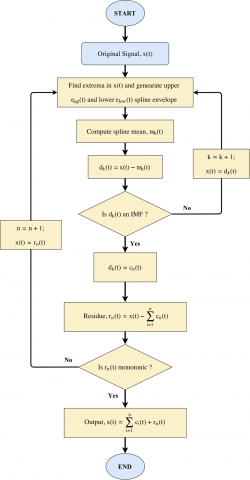Figure 1. EMD flowchart

3. Proposed Algorithm

In this work, we applied a new EMD-based detection technique for noise signal denoising and correlation for leak location. According to the algorithm:

1. Decomposition of the signal into IMFs.
2. Choose the IMFS containing the leak information by the experiment.
3. Application of the correlation the delay time.
4. Calculate the position of the leak with respect to one of the acoustic sensors; using the speed of sound in the air.
4. Experimental System

4.1 Experimental system synoptic

Our leak detection system is based mainly on the acoustic kit (measuring microphone and amplifier) for the capture and conditioning of analog audio signals, and on the STM32F4xx board for the acquisition and digitization of data before the treatment phase in a PC using numerical techniques. In this diagram, we present the different operating blocs used for the acquisition of sound Leakage signals (Figure 2).

## 2.pngFigure 2. Setup of the proposed acquisition system

4.2 Used materials

4.2.1 Acoustic sensor 03542-00 and the PHYWE amplifier

The measuring microphone is suitable for special acoustic soundings, for example, a dot plot of sound fields. Its three selectable output modes (Signal ~, Level, Trigger), built-in amplifier and free choice of power supply, i.e. battery or mains unit, allow it to be used universally (Figure 3).

## 3.pngFigure 3. a) Acoustic signal sensor (measurement microphone); b) Acoustic signal amplifier

4.2.2 Signal conditioning stage

Electrical signals from sensors are generally low level. If one wants to work with good precision, it is necessary to amplify them. But this amplification should only concern the wanted signal. This one often rubs a parasitic voltage (often of the same order of magnitude as the useful signal). So we have to do a selective amplification which eliminates or strongly attenuates any signal not containing information to keep only the sensor signal. Among the best circuits used to this task is the instrumentation amplifier (Figure 4). Its disadvantage is that it is expensive so we opted for a similar assembly, the adaptation montage is made of such so that it delivers a voltage of between 0 ≤ Vch1, ch2≤ 3V for the two signals delivered by the low-frequency amplifier. These two signals will directly attack the four and five (4-5) inputs of the STM32F4xx board.

## 4.pngFigure 4. Signal conditioner

4.2.3 STM32F4xx board

The STM32F4xx DISCOVERY kit allows users to easily develop applications with high performance from embedded microcontrollers in theSTM32F4xx module to ARM® Cortex®-M4 32-bit core. It designed for it to be used by beginners or experienced users directly exploitable by Matlab tool. The STM32F407VGT6, includes an ST-LINK/V2 or ST-LINK V2 is an integrated tool of debugging, two ST MEMS digital accelerometers, a digital microphone, a DAC built-in class D audio with driver for a speaker, LEDs, push buttons and a USB connector for a micro-AB OTG.

## 5.pngFigure 5. Complete experimental setup (a) hydraulic circuit; (b) Acquisition system

4.2.4 Experimental hydraulic plant prototype

The Hydraulic plant prototype for water leak-detection with a two acoustic sensor is presented in Figure 5.

5. Results and Discussion

Before starting the acquisition of our signals, a calibration of the localization system is necessary. For this purpose, we made the first test by depositing the sensors at a precise distance of 1m from the leak.

5.1 Location system calibration test

Calibration (both sensors are installed at a distance of 1 m from the leak). Normally with this arrangement, the application of the correlation algorithm brings me back to a distance of 1 m.

In this test, we installed the two sensors beforehand at a distance of 100 cm on either side of the leak to ensure that the distance after the application of the algorithm gives me the exact distance. So after the practical test, the two sensors showed us that the results obtained are similar to the chosen distances.

5.1.1 Channel one signal

As shown in Figure 6, the time signal is composed of two parts, one without leak taking the duration of 10s and the other with a leak, triggered in the middle of the acquisition time of signal channel one (CH1) taking the same period.

The same condition applied for the acquisition of the second signal channel two (CH2), and also to say that the two signals are acquired at the same time.

## 6.png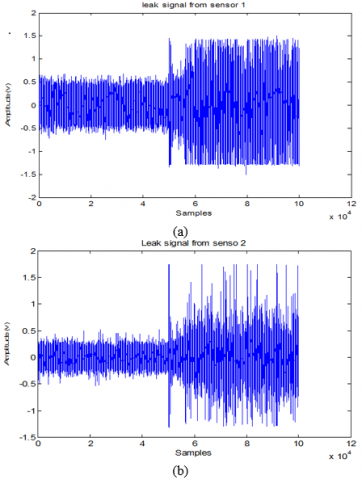Figure 6. Signal acquired: a) channel one, b) channel two

## 7a.png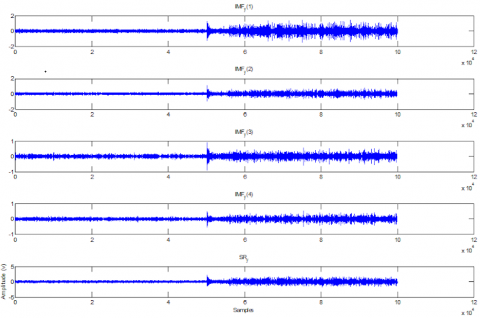(a)

## 7b.png(b)

Figure 7. Four IMFs and the SRy residus signals: a) CH1, b) CH2

5.1.2 Behavioral analysis of the different IMFs

After applying the EMD decomposition on the signal of the channel one (Figure 6.a), we obtained the signals of the four IMFs and the residus SRy (Figure 7), we can see that the first IMFy-1 and the IMFy-3 are very close to the signal. However, the IMFy-2 and IMFy-4 do not match the original signal.

After applying the EMD method to the signal from channel two (Figure 6.b), the signals from the four IMFs were obtained along with the residus SRs and the same interpretation as the IMF signals obtained from channel one. The cross-correlation of the two IMFS (IMFy-1 and IMFs-1) confirms the exact distance of 1m from one of the two sensors with respect to the leak.

## 8.pngFigure 8. Cross-correlation signal of the two IMFs (IMFy-1 & IMFs-1)

## 9.pngFigure 9. The spectrum of the signals: a) CH1, b) CH2

Figure 8 shows the exact position of one of the two sensors with respect to the leak (x = 0) that is to say that the sensor is a (1 m) one meter from the leak.

After execution of the program developed under Matlab for the determination of the time offset essentially for calculating the distance of the leak with respect to one of the sensors. The results show that the difference in time between the two signals is zero, $(\Delta \mathrm{t} \approx 0)$ so the distance from one of the two sensors equal to 100 cm (d = 1 m).

5.1.3 FFT of the signals of the two channels CH1 and CH2

By comparison of the two spectra of the Figures 9a and 9b, it can be seen that the second channel that the peaks found are virtually identical to that of channel one.

5.2 The second test

In this test, we placed the first sensor at a distance of $20 \mathrm{cm}$ and the second at a distance of $30 \mathrm{cm}$ from the leak. The sensors are oriented towards the leak. The signal of the channel one: as shown in Figure $10,$ a two-part signal is seen in the time window. The first without leakage and the other leaked. It is obvious that the leak is triggered in the middle of the time of acquisition of channel $(\mathrm{CH} 1)$

## 10.png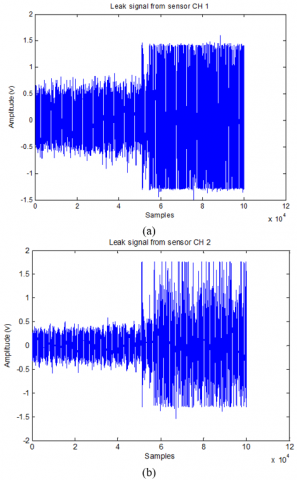Figure 10. Acquired signal: a) channel one, b) channel two

## 11a.png(a)

## 11b.png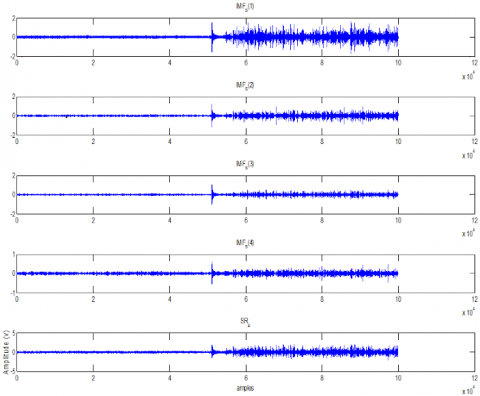(b)

Figure 11. The four IMFs and the SRy residus signals: a) CH1, b) CH2

Figures 11.a and 11.b show the four IMFs as well as the residus of the two channels CH1 and CH2, respectively. The correlation of the two signals (IMFy-1 and IMFs-1) gives the time to calculate the distance of one of the two sensors from the leak.

## 12.pngFigure 12. Cross-correlation signal of the two IMFs (IMFy-1& IMFs-1)

## 13a.png(a)

## 13b.png(b)

Figure 13. Spectrum signals of the two channels a) CH1, b) CH2

Figure 12 can give us the time difference between the two signals of the canal one and the two canals. This allows the calculation of the exact position of one of the two sensors in relation to the leak. In this case, we have Δt ≈ 0.5 ms, i.e. the sensor is at 0.33 m from the leak.

5.2.1 FFT signals of the two channels CH1 and CH2

By analyzing the spectra of the two IMFs of the two signals from the two channels, the information relating to the leak is between 2kHz and 3kHz (Figure 13).

5.3 The third test

Sensor (1) at a distance of 50Cm and the second sensor (2) at a distance of 90Cm of the leak. As it is represented in this Figures 14.a and 14.b, we see the signal is composed of two segments, one without leakage and the other with leakage. The leak is triggered in the middle of the time window of the signal acquisition time of channel one (CH1) and channel two (CH2). In this test, we placed the first sensor at a distance of 20 cm and the second at a distance of 30 cm from the leak. The sensors are oriented towards the leak. The signal of the channel one: as shown in the figure, a two-part signal is seen in the time window. The first without leakage and the other leaked. It is obvious that the leak is triggered in the middle of the time of acquisition of channel (CH1). In this last test, it is noted that the average values of the two acquired signals CH1 and CH2 decreases as the distance between the sensors and the leak increases.

## 14a.png(a)

## 14b.png(b)

Figure 14. Acquired signal: a) the channel one, b) the channel two

## 15a.png(a)

## 15b.png(b)

Figure 15. The four IMFs and the SRy residus signals: a) CH1, b) CH2

Figures 15.a and 15.b show the four IMFs as well as the residus of the two channels CH1 and CH2, respectively. The correlation of the two signals (IMFy -1 and IMFs -1) gives the time to calculate the distance of one of the two sensors from the leak; while Figure 16 can give us the time difference between the two signals of the canal one and the two canals. This allows the calculation of the exact position of one of the two sensors in relation to the leak. In this case, we have Δt≈10 ms, i.e. the sensor is at 0.87m from the leak.

## 16.pngFigure 16. Cross-correlation signal of the two IMFs (IMFy-1& IMFs-1)

## 17a.png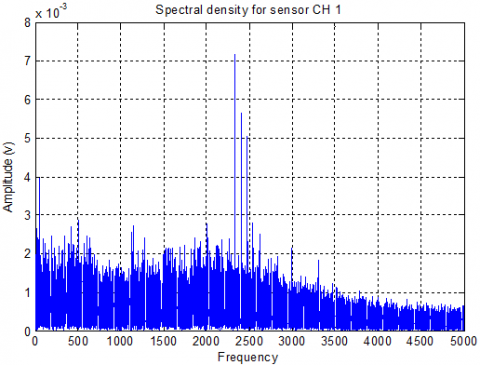(a)

## 17b.png(b)

Figure 17. Spectrum signals of the two channels: a) CH1, b) CH2

5.3.1 FFT of the signals of the two channels CH1 and CH2

Figure 17 shows the the spectrum of the signals of the two channels CH1 and CH2.

By analyzing the spectra of the two IMFs of the two signals from the two channels, the information relating to the leak is between 2 KHz and 3 KHz.

6. Conclusion

The work we have done in this paper focuses on the detection and location of leakage in a distribution network based on a fairly recent technique named EMD. This method, allows a non-stationary and non-linear signal to be decomposed into a single-component signal set called IMFs. It allows estimating the amplitude and the instantaneous frequency for each component. EMD is an innovative and more advantageous technique for the detection of signal singularities compared to other nonlinear and non-stationary signal analysis techniques like FFT, STFT, ...etc. This new technique EMD has been applied to the water distribution networks to be able to analyze the defects that occur on the latter. The analysis showed that the application of the first IMF for determining the position of the leak shows good efficiency, this is because the information is located in the first IMF. The tests that have been done have proved the effectiveness of the method used for choosing the IMF for locating the leak. The results obtained showed the accuracy of the proposed method. As perspective, we plan to develop an embedded system for the detection of leaks in water distribution networks.

References

 IEA – Water-Energy Nexus (2018).

 Majidi Khalilabad, N., Mollazadeh, M., Akbarpour, A., Khorashadizadeh, S. (2018). Leak detection in water distribution system using non-linear Kalman filter. International Journal of Optimization in Civil Engineering, 8(2): 169-180.

 Rahmat, R.F., Satria, S., Siregar, B., Budiarto, R. (2017). Water pipeline monitoring and leak detection using flow liquid meter sensor. IOP Conference Series: Materials Science and Engineering, 190. https://doi.org/10.1088/1757-899X/190/1/012036

 Blažević, M., Samardžić, I., Kolumbić, Z. (2005). Leak detection in underground pipelines of municipal water distribution. 4th DAAAM International Conference on Advanced Technologies for Developing Countries (ATDC’05), SlavonskiBrod, Croatia, pp. 481-486.

 Tang, X.H., Liu, Y.B., Zheng, L.J., Ma, C.B., Wang, H. (2010). Leak detection of water pipeline using wavelet transform and correlation method. J. Environ. Technol. Eng., 3(2): 62-67. https://doi.org/10.1109/ESIAT.2009.57

 Addison, P.S. (2005). Wavelet transforms and the ECG: a review. Physiol. Meas., 26(5): 155-199. https://doi.org/10.1088/0967-3334/26/5/R01

 Aggarwal, R., Singh, J.K., Gupta, V.K., Rathore, S., Tiwari, M., Khare, A. (2011). Noise reduction of speech signal using wavelet transform with modified universal threshold. Int. J. Comput. Appl., 20(5): 14-19. https://doi.org/10.5120/2431-3269

 Huang, N.E., Shen, Z., Long, S.R., Wu, M.C., Shih, H.H., Zheng, Q., Yen, N.C., Tung, C.C., Liu, HH. (1998). The empirical mode decomposition and the Hilbert spectrum for nonlinear and non-stationary time series analysis. Proceedings of the Royal Society of London Series, 454(1971): 903-995. https://doi.org/10.1098/rspa.1998.0193

 Santos, R.B., de Almeida, W.S., da Silva, F.V., da Cruz, S.L., Fileti, A.M.F. (2013). Spectral analysis for detection of leaks in pipes carrying compressed air. Chem. Eng. Trans, 32: 1363-1368. https://doi.org/10.3303/CET1332228

 Adedeji, K., Hamam, Y., Abe, B., Abu-Mahfouz, A.M.I. (2017). Leakage detection algorithm integrating water distribution networks hydraulic model. SimHydro 2017: Choosing the Right Model in Applied Hydraulics, Sophia Antipolis, France.

 Bentoumi, M. (2017). Wavelet DT method for water leak-detection using a vibration sensor: an experimental analysis. IET Signal Process Journal, 11(4): 396-405. https://dx.doi.org/10.1049/iet-spr.2016.0113

 Nasirian, A., Maghrebi, M.F., Yazdani, S. (2013). Leakage detection in water distribution network based on a new heuristic genetic algorithm model. J. Water Resour. Prot., 5(3): 294-303. https://doi.org/10.4236/jwarp.2013.53030

 Taghvaei, M., Beck, S.B.M., Staszewski, W.J. (2006). Leak detection in pipelines using cepstrum analysis. J. Meas. Sci. Technol., 17(2): 367-372. https://doi.org/10.1088/0957-0233/17/2/018

 Ghazali, M.F., Beck, S.B.M., Shucksmith, J.D., Boxall, J.B., Staszewski, W.J. (2012). Comparative study of instantaneous frequency-based methods for leak detection in pipeline networks. J. Mechanical Systems and Signal Processing, 29: 187-200. https://doi.org/10.1016/j.ymssp.2011.10.011

 Chuanhu, G.E., Yang, H.Y., Ye, H., Wang, G.Z. (2009). A fast leak locating method based on wavelet transform. J. Tsinghua Sci. Technol., 14(5): 551-555. https://doi.org/10.1016/S1007-0214(09)70116-6